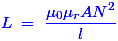# MCQs on Electromagnetic Fields

##### Page 16 of 20. Go to page 1 2 3 4 5 6 7 8 9 10 11 12 13 14 15 16 17 18 19 20
01․ The inductance of a long solenoid of length 1000 mm wound uniformly with 3000 turns on a cylindrical paper cable of 60 mm diameter is
3.2 µH.
3.2 mH.
32 mH.
3.2 H.

Inductor of solenoid is given as L = µ0N2A/l.

02․ The magnitude of force acting on a current carrying conductor placed in a magnetic field is independent of
flux density.
length of conductor.
current flowing through the conductor.
cross-sectional area of conductor.

F= BILsinθ so it independent of the cross-sectional area.

03․ Poyniting vector has the dimensions
Watt/metre.
Watts/metre2.
Watt × metre2.
Watt × metre.

Poynting vector represents the directional energy flux density of an electromagnetic field. So the dimension of poynting vector is watt per metre square.

04․ A medium is said to be isotropic when
∈ is zero.
∈ is a scalar constant.
∈ = μ.
∈ = μσ.

An isotropic medium is one such that the permittivity, permeability of the medium are uniform in all directions of the medium, the most simple instance being free space.

05․ If the resistance of a conductor does not vary in accordance with the Ohms law it is known as
non - conductor.
non - linear conductor.
reverse conductor.

Ohms law, V = IR which is an equation of straight line so the resistance of a conductor does not vary in accordance with the Ohms law it is known as non-linear conductor.

06․ Which of following is the unit of inductance?
Ampere turns.
Ohm.
Webers / m.
Milli- henry.

A Henry (H) is the derived SI unit of inductance. Henry is referred to the inductance of a circuit, in which changing of current with the rate of one ampere per second creates electromotive force of one volt. Then, we can write the Henry as milli - henry, micro - henry, nano - henry, pico- henry, etc.

07․ The core of coil length is 200 mm. The inductance of coil is 6 mH. If the core length is doubled, all other quantities, remaining the same, the inductance will be
3 mH.
12 mH.
24 mH.
48 mH.

The expression of inductance is given by,From this expression, we came to know that the inductance is inversely proportional to length of the core. Hence, if length gets increased by two, then by the same factor inductance would be decreased. So, the inductance value decreases from 6 mH to 3mH due to the increase of core length.

08․ For DC voltage an inductor is virtually a
short circuit.
open circuit.
depends on polarity.
depends on magnitude of voltage.

In DC supply, the current and voltage are constant. If we apply DC supply across an inductor, then there is no change in voltage and the current gradually increases, due to which the steady state voltage across the inductor is zero. Hence, it virtually acts as a short circuit.

09․ For an inductor, current is proportional to
magnetic field.
voltage across the inductor.
both (A) and (B).
none of them.

Each electron can move in a conductor that creates its own magnetic field. As electrons move through the coil of wire, the magnetic field of one electron adds to the field of any others moving in the same direction. Hence, the current should be directly proportional to magnetic field in an inductor.

10․ In an iron core coil the iron core is removed so that the coil becomes an air core coil. The inductance of the coil will
increase.
decrease.
remain the same.
initially increase and then decrease.

When the magnetic field creation is from the current carrying coils that goes through the iron core and that would line up with each magnetic field, it adds the total strength of magnetic field. So, if we remove that iron core, the line up action in each magnetic field does not happen. Hence, the inductance of the coil decreases.

<<<1415161718>>>